# Solution September 20, 2007

### Problem

Does there exist a sequence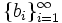$\{b_{i}\}_{i=1}^\infty$ of positive real numbers such that for each natural m: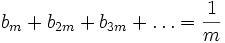$b_{m}+b_{2m}+b_{3m}+\dots=\frac1m$

### Solution

Assume that there is such a sequence (bi).

Let P be a set of prime numbers. Say that P divides n (symbolically, P | n) if any member of P divides n.

Define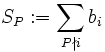$S_P := \sum_{P \nmid i}b_i$

SP is well-defined because it sums a subsequence of (bi) and all bi are positive.

Lemma: If P is finite, then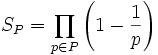$S_P = \prod_{p \in P} \left(1-\frac1p\right)$

Proof: By induction on the size of P. If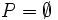$P=\empty$ then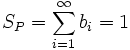$S_P = \sum_{i=1}^\infty b_i = 1$.

Now let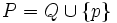$P = Q \cup\{p\}$ with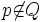$p\not\in Q$. Consider the sequence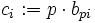$c_i := p\cdot b_{pi}$. It satisfies all requirements of (bi). Therefore,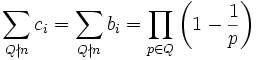$\sum_{Q\nmid n}c_i = \sum_{Q\nmid n} b_i = \prod_{p \in Q} \left(1-\frac1p\right)$.

A number n satisfies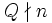$Q\nmid n$ if either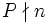$P\nmid n$ or p | n and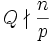$Q\nmid \frac np$. So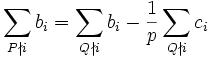$\sum_{P\nmid i} b_i = \sum_{Q\nmid i} b_i - \frac1p\sum_{Q\nmid i} c_i$

and we get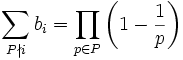$\sum_{P\nmid i} b_i = \prod_{p \in P} \left(1-\frac1p\right)$

This completes the induction step and thus proves the lemma.

Let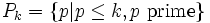$P_k = \{p | p \leq k, p \mathrm{\ prime}\}$.

Because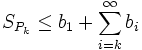$S_{P_k} \leq b_1 + \sum_{i=k}^\infty b_i$ we have that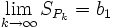$\lim_{k\to\infty}S_{P_k} = b_1$

Therefore,$b_1 = \prod_{p \mathrm{\ prime}}\left(1-\frac1p\right) = 0$

which is not positive. Thus no sequence (bi) satisfying the problem's requirements exists.

Note: By considering$c_i := k\cdot b_{ki}$ we can show that bk = 0 for all k. So the answer does not change if we replace "positive" by "nonnegative".

### Short "proof"

This "proof" makes use of the Möbius function (http://mathworld.wolfram.com/MoebiusFunction.html).

Assume there is such a sequence (bi). Let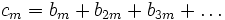$c_m = b_{m}+b_{2m}+b_{3m}+\dots$

Consider the sum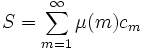$S = \sum_{m=1}^\infty \mu(m)c_{m}$

Now on the one hand,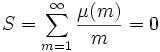$S = \sum_{m=1}^\infty \frac{\mu(m)}m = 0$

On the other hand,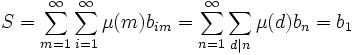$S = \sum_{m=1}^\infty\sum_{i=1}^\infty \mu(m)b_{im} = \sum_{n=1}^\infty\sum_{d|n}\mu(d)b_{n} = b_1\,$

So b1 = 0 which doesn't satisfy the requirements of the problem.